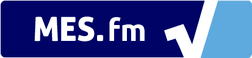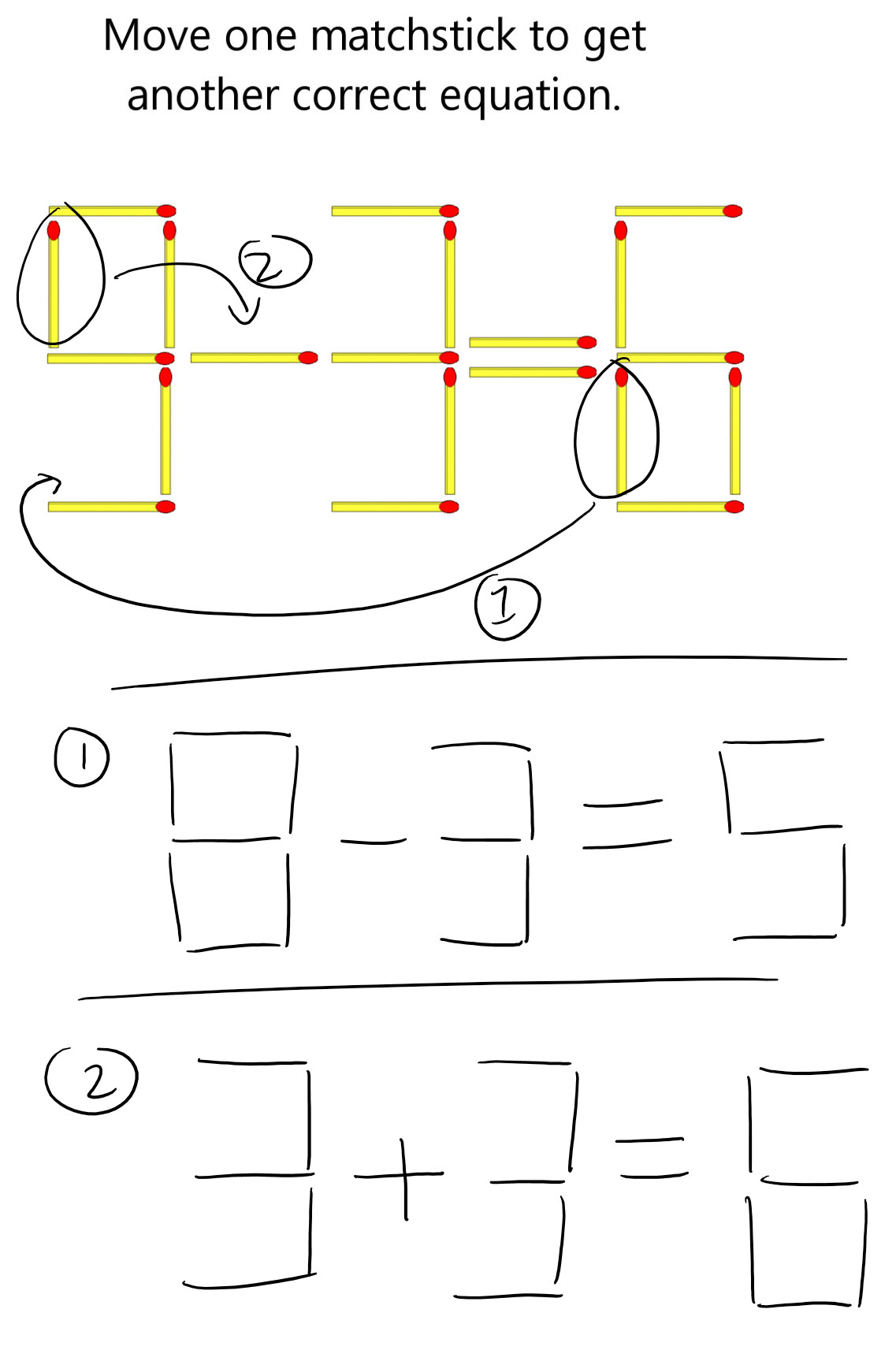We notice that you are using AdBlock. Please whitelist Math Easy Solutions to help keep this site up and running! :)# Match Stick Equation Puzzle (SOLUTION)

Here are two possible solutions to this very interesting match stick math puzzle.

The first solution involves moving the match stick from the number 6 to the number 9 and thus creating the equation 8 - 3 = 5.

The second solution is to move the match stick from the number 9 and moving it ontop of the minus sign to create the equation 3 + 3 = 6.

Can you think of any more solutions?

Check out the puzzle (without the solution) by clicking here!